# Derivation of Neoclassical Fluid Equations

It is helpful to define the so-called magnetohydrodynamical (MHD) velocity ,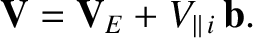(2.321)

which is the fluid velocity associated with the ion guiding centers. Here, a guiding center is the imaginary point about which a charged particle gyrates in the plane perpendicular to the local magnetic field. According to Section 2.11, the lowest-order ion and electron fluid velocities can be written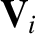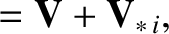(2.322)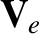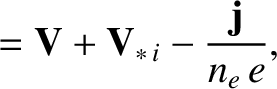(2.323)

respectively, where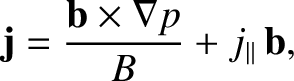(2.324)

is the lowest-order electrical current density. Quasi-neutrality demands that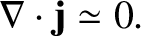(2.325)

The electron and ion continuity equations, (2.26) and (2.29), can be combined with Equations (2.322), (2.323), and (2.325) to give an electron number density continuity equation of the form :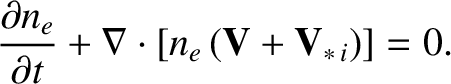(2.326)

If we sum the electron and the ion equations of motion, (2.27) and (2.30), then we obtain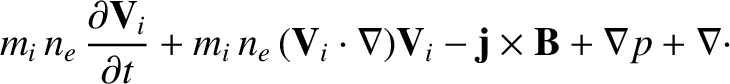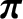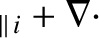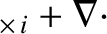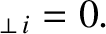(2.327)

Here,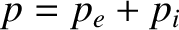is the total plasma pressure, and use has been made of Equations (2.16), (2.39), and (2.59). In the previous equation, we have neglected electron inertia with respect to ion inertia, because the former is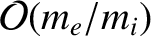times smaller than the latter. We have also neglected electron viscosity with respect to ion viscosity, because the former is, at least,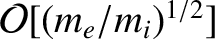times smaller than the latter. Now, it can be demonstrated that [28,29]: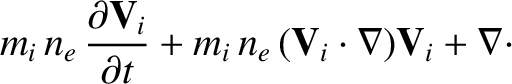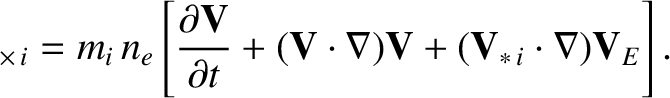(2.328)

This important result is known as the gyro-viscous cancellation. Furthermore, it is clear from Equations (2.152) and (2.161) that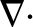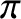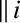acts predominately in the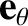direction (i.e., parallel to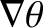). A model form forthat is consistent with the analysis of Section 2.18 is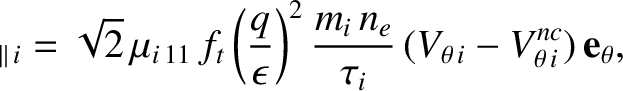(2.329)

where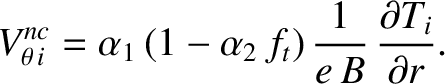(2.330)

Thus, the neoclassical ion parallel viscosity tensor acts to relax the ion poloidal velocity toward the neoclassical velocity specified in Equation (2.330) on a timescale that is roughly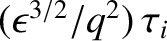. As has already been mentioned, this effect is known as poloidal flow damping. Equations (2.328) and (2.329) can be combined with Equation (2.303) to give an MHD equation of motion of the form: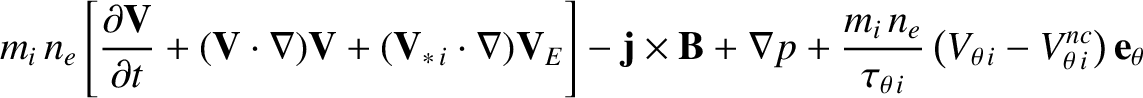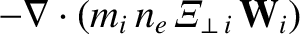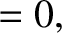(2.331)

where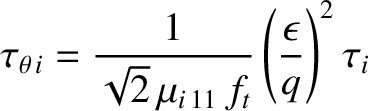(2.332)

is the poloidal flow-damping time. Of course, the value of the anomalous perpendicular momentum diffusivity,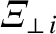, must be taken from experiments.

The electron equation of motion, (2.27), can be combined with Equations (2.59), (2.139) and (2.323) to give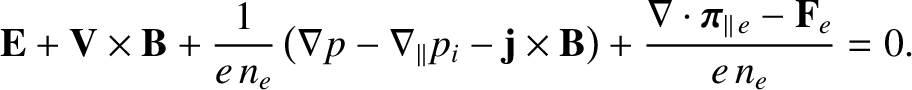(2.333)

Here, we have neglected electron inertia and gyro-viscosity, because these terms are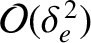times smaller than the leading-order terms. We have also neglected anomalous electron perpendicular viscosity because there are no available experimental measurements of this effect in tokamak plasmas. Let us adopt the following model form for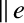which is consistent with the analysis of Sections 2.16, 2.19, and 2.20: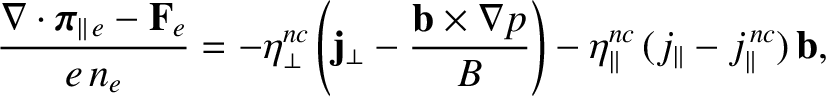(2.334)

where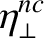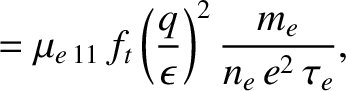(2.335)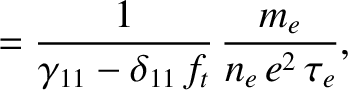(2.336)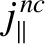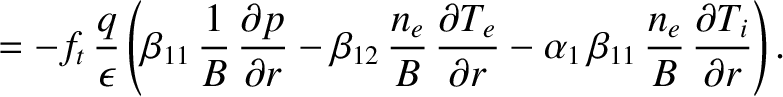(2.337)

Thus, the neoclassical electron parallel viscosity tensor acts to relax the perpendicular current to its lowest-order value [see Equation (2.324)], enhances the parallel electrical resistivity of the plasma, and also generates the non-inductive parallel current specified in Equation (2.337). As has already been mentioned, this non-inductive current is known as the bootstrap current. Note that in Equation (2.334) we have neglected the parallel thermal force density, (2.306), in accordance with the discussion in Section 2.23. We have also neglected the perpendicular component of the thermal force density, (2.307), because it is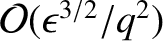smaller than the similar term in the previous equation that involves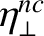. Thus, Equations (2.333) and (2.334) yield the following generalized Ohm's law for the plasma: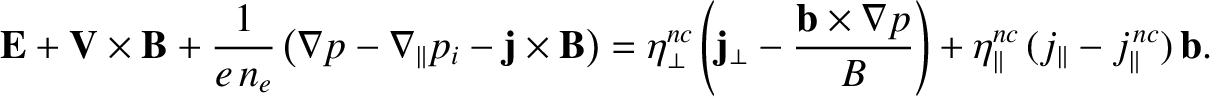(2.338)

The electron and ion energy conservation equations, (2.28) and (2.31), yield the following electron and ion energy conservation equations: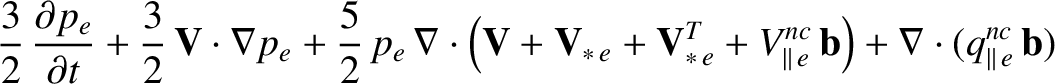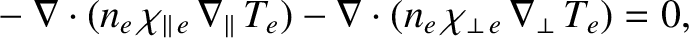(2.339)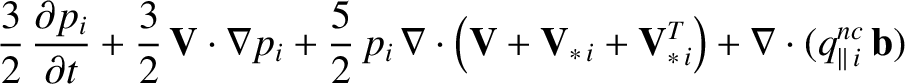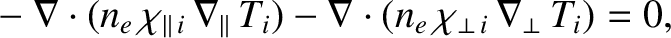(2.340)

where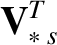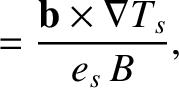(2.341)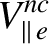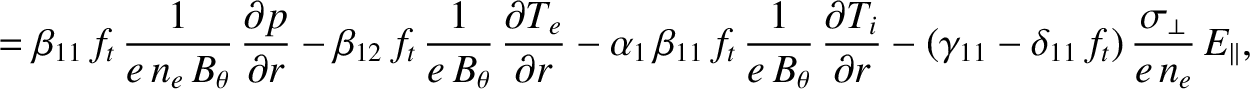(2.342)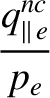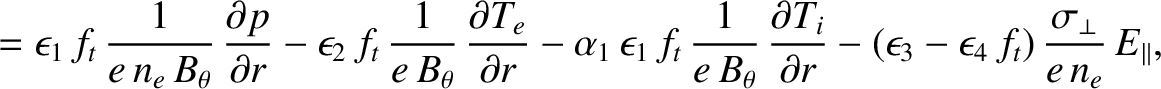(2.343)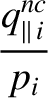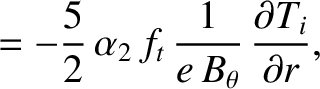(2.344)

and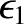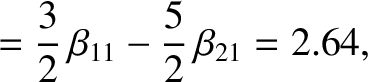(2.345)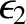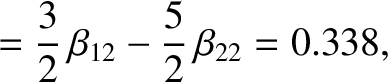(2.346)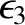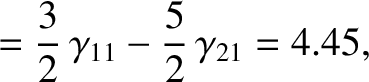(2.347)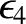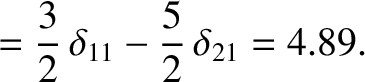(2.348)

In writing Equations (2.339) and (2.258), use has been made of Equations  (2.139), (2.226)–(2.229), (2.259)–(2.262), (2.322), and (2.323). We have neglected electron-ion energy exchange (i.e.,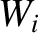), because this is a small effect, due to the smallness of the mass ratio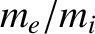. [See Equation (2.37).] We have also neglected ohmic heating (i.e.,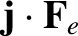), because this effect is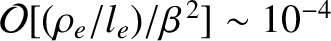(see Tables 1.2 and 2.1) smaller than the leading order terms. In addition, we have neglected viscous heating, because this effect is, at least,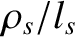times smaller than the leading-order terms. In accordance with the discussion in Section 2.23, we have neglected the parallel component of the thermal heat flux, (2.47). We have also neglected the perpendicular component of the thermal heat flux because this term is the same size as the classical electron perpendicular heat flux (and, therefore, much smaller than the anomalous electron perpendicular heat flux). The parallel heat “diffusivities”,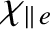and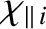, are given the collisionless values specified in Equations (2.319) and (2.320), respectively. Of course, the values of the anomalous perpendicular heat diffusivities,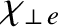and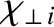, must be taken from experiments.

Note, finally, that when combined with the following subset of Maxwell's equations,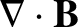(2.349)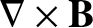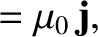(2.350)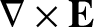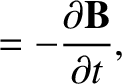(2.351)

our final set of neoclassical fluid equations, (2.326), (2.331), and (2.338)–(2.340), form a complete set.